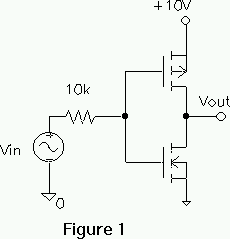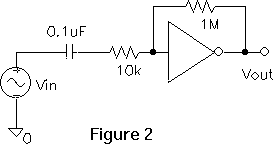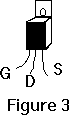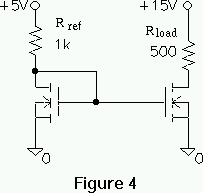EE321 Lab #12

MOSFET II

Prelab for Lab 12

The purpose of this lab is test several MOSFET circuits The CMOS 4007 integrated circuit contains 6 MOSFET's 3 n-channel and 3 p-channel. The n-channel bodies are connected to pin 7 and must be kept at the most negative voltage used in the circuit. The p-channel bodies are connected to pin 14 and must be kept at the most positive voltage used in the circuit. The drain and source are interchangeable on Q2 and Q5.

Anti-static precautions must be taken!

1. Build the CMOS inverter (Figure 1). The input should be preset to go from 0 to 10 V. The input resistor protects diode junctions that would be forward biased if the input exceeds its range.

• Test the circuit and copy the transfer curve. label the axis with the proper units. It will be similar to that in figure 5.58 in Sedra and Smith.
• Estimate the slope at its maximum.2. Add a feedback resistor and input capacitor to make a small signal amplifier (Figure 2). The large resistor causes the input and output to be equal at low frequencies causing the bias to be in the center of steep portion of the transfer curve, at the point of high gain. The feedback is not enough to effect the mid frequency gain.

• Measure the DC bias voltages.
• Estimate the output bias from the transfer curve you just measured.

3. Test the circuit to find the small signal gain.

• Measure the gain.
• Estimate the gain from part 1.
• How large can the output be and still be mostly undistorted?

MOSFET POWER SWITCH

4. Design build and test a MOSFET circuit turns on and off a lamp.

• Use a 20 watt power n-channel MOSFET (IRF711 rated at 350 V and 10 A, Figure 3).
• The lamp is rated at 5 V and draws 60 mA.
• The input should be a 0.2 Hz square wave 0 to 5 V.5. Connect the input to keep the lamp on with 5 V across it.

• Measure the vDS.
• What is the on resistance of the MOSFET. (assume the lamp draws its rated current).
• Estimate the power dissipated by the MOSFET.
• How large (watts) of a lamp could this MOSFET turn on?

CURRENT MIRROR

6. Build and test the current mirror shown in Figure 4, using a 4007.• The reference current is determined by Rref. Measure the voltage across Rref and Rload and confirm that the currents are about the same.
• Extra Credit. Decrease the +15V supply to +10V. Determine Rout (Norton equivalent circuit) from the small change in current.